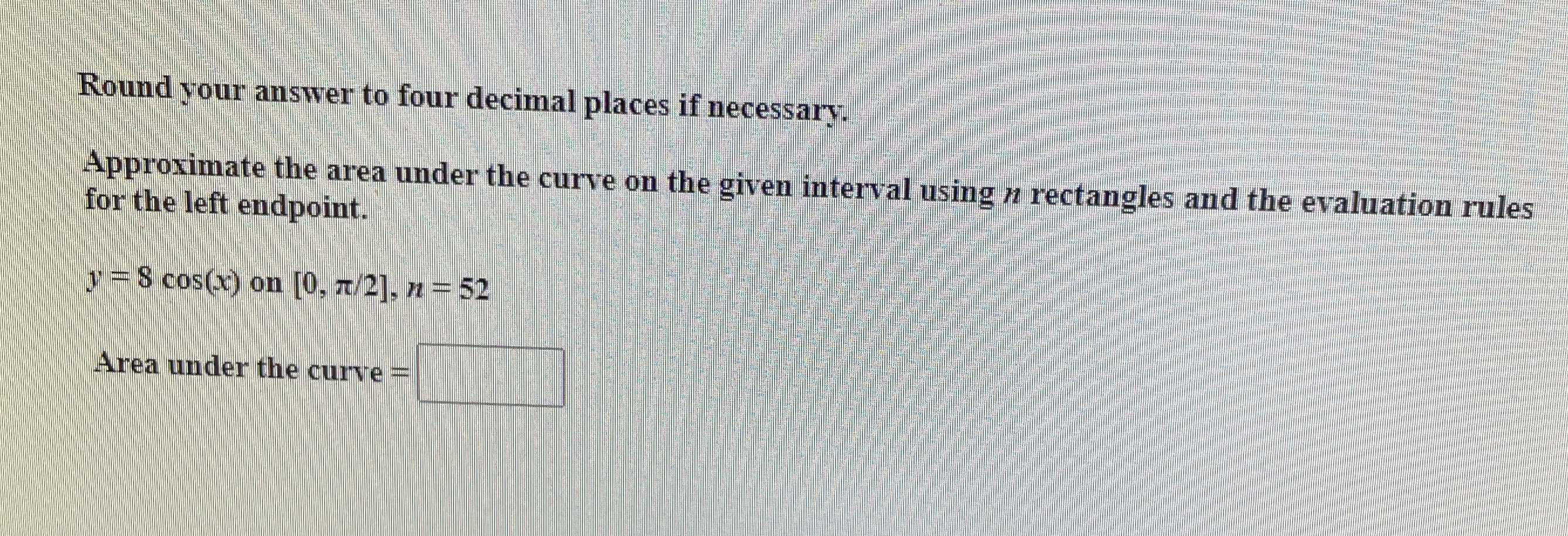### Still have math questions?

Trigonometry
QuestionRound your answer to four decimal places if necessary. Approximate the area under the curve on the given interval using $$n$$ rectangles and the evaluation rules for the left endpoint.

$$y = 8 \cos ( x )$$ on $$[ 0 , \pi / 2 ] , n = 52$$

Area under the curve